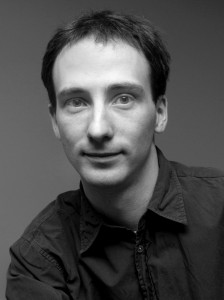# Peter Selinger – Number-theoretic methods in quantum computing

#### April 28th, 2016An important problem in quantum computing is the so-called approximate synthesis problem: to find a quantum circuit, preferably as short as possible, that approximates a given unitary operator up to given epsilon. Moreover, the solution should be computed by an efficient algorithm. For nearly two decades, the standard solution to this problem was the Solovay-Kitaev algorithm, which is based on geometric ideas. This algorithm produces circuits of size O(log^c(1/epsilon)), where c is approximately 3.97.

It was a long-standing open problem whether this exponent c could be reduced to 1. In this talk, I will report on a number-theoretic algorithm that achieves circuit size O(log(1/epsilon)) in the case of the so-called Clifford+T gate set, thereby answering the above question positively.

In case the operator to be approximated is diagonal, the algorithm satisfies an even stronger property: it computes the optimal solution to the given approximation problem. The algorithm also generalizes to certain other gate sets arising from number-theoretic unitary groups. This is joint work with Neil J. Ross.

Bio: Peter Selinger is a Professor of Mathematics and Computer Science at Dalhousie University. He received his Ph.D. is from the University of Pennsylvania in 1997. His main research interest is the semantics of programming languages, and specifically the theory of programming languages for quantum computing, which he helped pioneer. More recently, he also became interested in the application of number-theoretic methods to unitary approximation problems. He is an editor of the journal Logical Methods in Computer Science and a founder of the workshop series Quantum Physics and Logic.

Les commentaires sont clos.